# The GENMOD Procedure

### BAYES Statement

• BAYES <options> ;

The BAYES statement requests a Bayesian analysis of the regression model by using Gibbs sampling. The Bayesian posterior samples (also known as the chain) for the regression parameters are not tabulated. The Bayesian posterior samples (also known as the chain) for the regression parameters can be output to a SAS data set. Table 43.2 summarizes the options available in the BAYES statement.

Table 43.2: BAYES Statement Options

Option

Description

Monte Carlo Options

Specifies the initial values of the chain

Specifies that maximum likelihood estimates be used as initial values of the chain

Specifies the use of a Metropolis step in the ARMS algorithm

Specifies the number of burn-in iterations

Specifies the number of iterations after burn-in

Specifies the algorithm used to sample the posterior distribution

Specifies the random number generator seed

Controls the thinning of the Markov chain

Model and Prior Options

Specifies the prior of the regression coefficients

Specifies the prior of the dispersion parameter

Specifies the prior of the precision parameter

Specifies the prior of the scale parameter

Summary Statistics and Convergence Diagnostics

Displays convergence diagnostics

Displays diagnostic plots

Displays summary statistics of the posterior samples

Posterior Samples

Names a SAS data set for the posterior samples

The following list describes these options and their suboptions.

COEFFPRIOR=JEFFREYS<(option)> | NORMAL<(options)> | UNIFORM
COEFF=JEFFREYS<(options)> | NORMAL<(options)> | UNIFORM
CPRIOR=JEFFREYS<(options)> | NORMAL<(options)> | UNIFORM

specifies the prior distribution for the regression coefficients. The default is COEFFPRIOR=UNIFORM, which specifies the noninformative and improper prior of a constant.

Jeffreys’ prior is specified by COEFFPRIOR=JEFFREYS, which can be followed by the following option in parentheses. Jeffreys’ prior is proportional to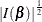, where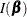is the Fisher information matrix. See the section Jeffreys’ Prior and Ibrahim and Laud (1991) for more details.

CONDITIONAL

specifies that the Jeffreys’ prior, conditional on the current Markov chain value of the generalized linear model precision parameter, is proportional to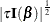.

The normal prior is specified by COEFFPRIOR=NORMAL, which can be followed by one of the following options enclosed in parentheses. However, if you do not specify an option, the normal prior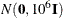, whereis the identity matrix, is used. See the section Normal Prior for more details.

CONDITIONAL

specifies that the normal prior, conditional on the current Markov chain value of the generalized linear model precision parameter, is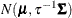, where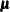and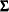are the mean and covariance of the normal prior specified by other normal options.

INPUT=SAS-data-set

specifies a SAS data set containing the mean and covariance information of the normal prior. The data set must have a _TYPE_ variable to represent the type of each observation and a variable for each regression coefficient. If the data set also contains a _NAME_ variable, the values of this variable are used to identify the covariances for the _TYPE_=’COV’ observations; otherwise, the _TYPE_=’COV’ observations are assumed to be in the same order as the explanatory variables in the MODEL statement. PROC GENMOD reads the mean vector from the observation with _TYPE_=’MEAN’ and reads the covariance matrix from observations with _TYPE_=’COV’. For an independent normal prior, the variances can be specified with _TYPE_=’VAR’; alternatively, the precisions (inverse of the variances) can be specified with _TYPE_=’PRECISION’.

RELVAR<=c>

specifies the normal prior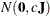, where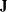is a diagonal matrix with diagonal elements equal to the variances of the corresponding ML estimator. By default,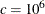.

VAR<=c>

specifies the normal prior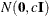, whereis the identity matrix.

DIAGNOSTICS=ALL | NONE | (keyword-list)
DIAG=ALL | NONE | (keyword-list)

controls the number of diagnostics produced. You can request all the following diagnostics by specifying DIAGNOSTICS=ALL. If you do not want any of these diagnostics, specify DIAGNOSTICS=NONE. If you want some but not all of the diagnostics, or if you want to change certain settings of these diagnostics, specify a subset of the following keywords. The default is DIAGNOSTICS=(AUTOCORR ESS GEWEKE).

AUTOCORR <(LAGS= numeric-list)>

computes the autocorrelations of lags given by LAGS= list for each parameter. Elements in the list are truncated to integers and repeated values are removed. If the LAGS= option is not specified, autocorrelations of lags 1, 5, 10, and 50 are computed for each variable. See the section Autocorrelations in Chapter 7: Introduction to Bayesian Analysis Procedures, for details.

ESS

computes Carlin’s estimate of the effective sample size, the correlation time, and the efficiency of the chain for each parameter. See the section Effective Sample Size in Chapter 7: Introduction to Bayesian Analysis Procedures, for details.

GELMAN <(gelman-options)>

computes the Gelman and Rubin convergence diagnostics. You can specify one or more of the following gelman-options:

NCHAIN | N=number

specifies the number of parallel chains used to compute the diagnostic, and must be 2 or larger. The default is NCHAIN=3. If an INITIAL= data set is used, NCHAIN defaults to the number of rows in the INITIAL= data set. If any number other than this is specified with the NCHAIN= option, the NCHAIN= value is ignored.

ALPHA=value

specifies the significance level for the upper bound. The default is ALPHA=0.05, resulting in a 97.5% bound.

See the section Gelman and Rubin Diagnostics in Chapter 7: Introduction to Bayesian Analysis Procedures, for details.

GEWEKE <(geweke-options)>

computes the Geweke spectral density diagnostics, which are essentially a two-sample t test between the first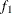portion and the last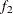portion of the chain. The default is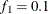and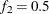, but you can choose other fractions by using the following geweke-options:

FRAC1=value

specifies the fractionfor the first window.

FRAC2=value

specifies the fractionfor the second window.

See the section Geweke Diagnostics in Chapter 7: Introduction to Bayesian Analysis Procedures, for details.

HEIDELBERGER <(heidel-options)>

computes the Heidelberger and Welch diagnostic for each variable, which consists of a stationarity test of the null hypothesis that the sample values form a stationary process. If the stationarity test is not rejected, a halfwidth test is then carried out. Optionally, you can specify one or more of the following heidel-options:

SALPHA=value

specifies the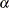level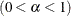for the stationarity test.

HALPHA=value

specifies thelevelfor the halfwidth test.

EPS=value

specifies a positive numbersuch that if the halfwidth is less thantimes the sample mean of the retained iterates, the halfwidth test is passed.

See the section Heidelberger and Welch Diagnostics in Chapter 7: Introduction to Bayesian Analysis Procedures, for details.

MCSE
MCERROR

computes the Monte Carlo standard error for each parameter. The Monte Caro standard error, which measures the simulation accuracy, is the standard error of the posterior mean estimate and is calculated as the posterior standard deviation divided by the square root of the effective sample size. See the section Standard Error of the Mean Estimate in Chapter 7: Introduction to Bayesian Analysis Procedures, for details.

RAFTERY<(raftery-options)>

computes the Raftery and Lewis diagnostics that evaluate the accuracy of the estimated quantile (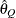for a given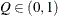) of a chain.can achieve any degree of accuracy when the chain is allowed to run for a long time. A stopping criterion is when the estimated probability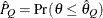reaches within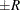of the value Q with probability S; that is,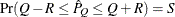. The following raftery-options enable you to specify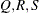, and a precision levelfor the test:

QUANTILE | Q=value

specifies the order (a value between 0 and 1) of the quantile of interest. The default is 0.025.

ACCURACY | R=value

specifies a small positive number as the margin of error for measuring the accuracy of estimation of the quantile. The default is 0.005.

PROBABILITY | S=value

specifies the probability of attaining the accuracy of the estimation of the quantile. The default is 0.95.

EPSILON | EPS=value

specifies the tolerance level (a small positive number) for the stationary test. The default is 0.001.

See the section Raftery and Lewis Diagnostics in Chapter 7: Introduction to Bayesian Analysis Procedures, for details.

DISPERSIONPRIOR=GAMMA<(options)> | IGAMMA<(options)> | IMPROPER
DPRIOR=GAMMA<(options)> | IGAMMA<(options)> | IMPROPER

specifies that Gibbs sampling be performed on the generalized linear model dispersion parameter and the prior distribution for the dispersion parameter, if there is a dispersion parameter in the model. For models that do not have a dispersion parameter (the Poisson and binomial), this option is ignored. Note that you can specify Gibbs sampling on either the dispersion parameter, the scale parameter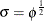, or the precision parameter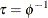, with the DPRIOR=, SPRIOR=, and PPRIOR= options, respectively. These three parameters are transformations of one another, and you should specify Gibbs sampling for only one of them.

A gamma prior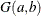with density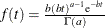is specified by DISPERSIONPRIOR=GAMMA, which can be followed by one of the following gamma-options enclosed in parentheses. The hyperparameters a and b are the shape and inverse-scale parameters of the gamma distribution, respectively. See the section Gamma Prior for details. The default is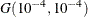.

RELSHAPE<=c>

specifies independent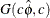distribution, where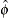is the MLE of the dispersion parameter. With this choice of hyperparameters, the mean of the prior distribution isand the variance is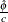. By default, c=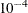.

SHAPE=a
ISCALE=b

when both specified, results in aprior.

SHAPE=c

when specified alone, results in a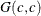prior.

ISCALE=c

when specified alone, results in aprior.

An inverse gamma prior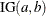with density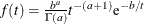is specified by DISPERSIONPRIOR=IGAMMA, which can be followed by one of the following inverse gamma-options enclosed in parentheses. The hyperparameters a and b are the shape and scale parameters of the inverse gamma distribution, respectively. See the section Inverse Gamma Prior for details. The default is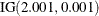.

RELSHAPE<=c>

specifies independent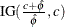distribution, whereis the MLE of the dispersion parameter. With this choice of hyperparameters, the mean of the prior distribution is. By default, c=.

SHAPE=a
SCALE=b

when both specified, results in aprior.

SHAPE=c

when specified alone, results in an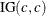prior.

SCALE=c

when specified alone, results in anprior.

An improper prior with density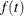proportional to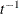is specified with DISPERSIONPRIOR=IMPROPER.

INITIAL=SAS-data-set

specifies the SAS data set that contains the initial values of the Markov chains. The INITIAL= data set must contain all the variables of the model. You can specify multiple rows as the initial values of the parallel chains for the Gelman-Rubin statistics, but posterior summaries, diagnostics, and plots are computed only for the first chain. If the data set also contains the variable _SEED_, the value of the _SEED_ variable is used as the seed of the random number generator for the corresponding chain.

INITIALMLE

specifies that maximum likelihood estimates of the model parameters be used as initial values of the Markov chain. If this option is not specified, estimates of the mode of the posterior distribution obtained by optimization are used as initial values.

METROPOLIS=YES | NO

specifies the use of a Metropolis step to generate Gibbs samples for posterior distributions that are not log concave. The default value is METROPOLIS=YES.

NBI=number

specifies the number of burn-in iterations before the chains are saved. The default is 2000.

NMC=number

specifies the number of iterations after the burn-in. The default is 10000.

OUTPOST=SAS-data-set
OUT=SAS-data-set

names the SAS data set that contains the posterior samples. See the sections OUTPOST= Output Data Set and Posterior Samples Output Data Set for more information. Alternatively, you can create the output data set by specifying an ODS OUTPUT statement as follows:

ODS OUTPUT POSTERIORSAMPLE=SAS-data-set

PRECISIONPRIOR=GAMMA<(options)> | IMPROPER
PPRIOR=GAMMA<(options)> | IMPROPER

specifies that Gibbs sampling be performed on the generalized linear model precision parameter and the prior distribution for the precision parameter, if there is a precision parameter in the model. For models that do not have a precision parameter (the Poisson and binomial), this option is ignored. Note that you can specify Gibbs sampling on either the dispersion parameter, the scale parameter, or the precision parameter, with the DPRIOR=, SPRIOR=, and PPRIOR= options, respectively. These three parameters are transformations of one another, and you should specify Gibbs sampling for only one of them.

A gamma priorwith densityis specified by PRECISIONPRIOR=GAMMA, which can be followed by one of the following gamma-options enclosed in parentheses. The hyperparameters a and b are the shape and inverse-scale parameters of the gamma distribution, respectively. See the section Gamma Prior for details. The default is.

RELSHAPE<=c>

specifies independent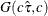distribution, whereis the MLE of the dispersion parameter. With this choice of hyperparameters, the mean of the prior distribution isand the variance is. By default,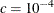.

SHAPE=a
ISCALE=b

when both specified, results in aprior.

SHAPE=c

when specified alone, results in anprior.

ISCALE=c

when specified alone, results in anprior.

An improper prior with densityproportional tois specified with PRECISIONPRIOR=IMPROPER.

PLOTS<(global-plot-options)>=plot-request
PLOTS<(global-plot-options)>=(plot-request < …plot-request>)

controls the display of diagnostic plots. Three types of plots can be requested: trace plots, autocorrelation function plots, and kernel density plots. By default, the plots are displayed in panels unless the global-plot-option UNPACK is specified. Also, when you are specifying more than one type of plots, the plots are displayed by parameters unless the global-plot-option GROUPBY is specified. When you specify only one plot-request, you can omit the parentheses around the plot-request. For example:

 plots=none
plots(unpack)=trace
plots=(trace autocorr)


ODS Graphics must be enabled before requesting plots. For example, the following SAS statements enable ODS Graphics:

ods graphics on;
proc genmod;
model y=x;
bayes plots=trace;
run;
ods graphics off;


The global-plot-options are as follows:

FRINGE

creates a fringe plot on the X axis of the density plot.

GROUPBY=PARAMETER
GROUPBY=TYPE

specifies how the plots are grouped when there is more than one type of plot.

GROUPBY=TYPE

specifies that the plots be grouped by type.

GROUPBY=PARAMETER

specifies that the plots be grouped by parameter.

GROUPBY=PARAMETER is the default.

LAGS=n

specifies that autocorrelations be plotted up to lag n. If this option is not specified, autocorrelations are plotted up to lag 50.

SMOOTH

displays a fitted penalized B-spline curve for each trace plot.

UNPACKPANEL
UNPACK

specifies that all paneled plots be unpacked, meaning that each plot in a panel is displayed separately.

The plot-requests include the following:

ALL

specifies all types of plots. PLOTS=ALL is equivalent to specifying PLOTS=(TRACE AUTOCORR DENSITY).

AUTOCORR

displays the autocorrelation function plots for the parameters.

DENSITY

displays the kernel density plots for the parameters.

NONE

suppresses all diagnostic plots.

TRACE

displays the trace plots for the parameters. See the section Visual Analysis via Trace Plots in Chapter 7: Introduction to Bayesian Analysis Procedures, for details.

SAMPLING=option

specifies an algorithm used to sample the posterior distribution. The following options are available:

ARMS
GIBBS

use the ARMS algorithm.

GAMERMAN
GAM

use the Gamerman algorithm. This is the default method except for the normal distribution with a conjugate prior. In this case a closed form for the posterior distribution is available, and samples are obtained directly from the posterior distribution.

IM

Use the independent Metropolis algorithm.

SCALEPRIOR=GAMMA<(options)> | IMPROPER
SPRIOR=GAMMA<(options)> | IMPROPER

specifies that Gibbs sampling be performed on the generalized linear model scale parameter and the prior distribution for the scale parameter, if there is a scale parameter in the model. For models that do not have a scale parameter (the Poisson and binomial), this option is ignored. Note that you can specify Gibbs sampling on either the dispersion parameter, the scale parameter, or the precision parameter, with the DPRIOR=, SPRIOR=, and PPRIOR= options, respectively. These three parameters are transformations of one another, and you should specify Gibbs sampling for only one of them.

A gamma priorwith densityis specified by SCALEPRIOR=GAMMA, which can be followed by one of the following gamma-options enclosed in parentheses. The hyperparameters a and b are the shape and inverse-scale parameters of the gamma distribution, respectively. See the section Gamma Prior for details. The default is.

RELSHAPE<=c>

specifies independent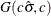distribution, where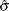is the MLE of the dispersion parameter. With this choice of hyperparameters, the mean of the prior distribution isand the variance is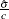. By default,.

SHAPE=a
ISCALE=b

when both specified, results in aprior.

SHAPE=c

when specified alone, results in anprior.

ISCALE=c

when specified alone, results in anprior.

An improper prior with densityproportional tois specified with SCALEPRIOR=IMPROPER.

SEED=number

specifies an integer seed in the range 1 to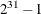for the random number generator in the simulation. Specifying a seed enables you to reproduce identical Markov chains for the same specification. If the SEED= option is not specified, or if you specify a nonpositive seed, a random seed is derived from the time of day.

STATISTICS <(global-options)> = ALL | NONE | keyword | (keyword-list)
STATS <(global-options)> = ALL | NONE | keyword | (keyword-list)

controls the number of posterior statistics produced. Specifying STATISTICS=ALL is equivalent to specifying STATISTICS= (SUMMARY INTERVAL COV CORR). If you do not want any posterior statistics, you specify STATISTICS=NONE. The default is STATISTICS=(SUMMARY INTERVAL). See the section Summary Statistics in Chapter 7: Introduction to Bayesian Analysis Procedures, for details. The global-options include the following:

ALPHA=numeric-list

controls the probabilities of the credible intervals. The ALPHA= values must be between 0 and 1. Each ALPHA= value produces a pair of 100(1–ALPHA)% equal-tail and HPD intervals for each parameters. The default is the value of the ALPHA= option in the MODEL statement, or 0.05 if that option is not specified (yielding the 95% credible intervals for each parameter).

PERCENT=numeric-list

requests the percentile points of the posterior samples. The PERCENT= values must be between 0 and 100. The default is PERCENT=25, 50, 75, which yield the 25th, 50th, and 75th percentile points, respectively, for each parameter.

The list of keywords includes the following:

CORR

produces the posterior correlation matrix.

COV

produces the posterior covariance matrix.

SUMMARY

produces the means, standard deviations, and percentile points for the posterior samples. The default is to produce the 25th, 50th, and 75th percentile points, but you can use the global PERCENT= option to request specific percentile points.

INTERVAL

produces equal-tail credible intervals and HPD intervals. The default is to produce the 95% equal-tail credible intervals and 95% HPD intervals, but you can use the global ALPHA= option to request intervals of any probabilities.

THINNING=number
THIN=number

controls the thinning of the Markov chain. Only one in every k samples is used when THINNING=k, and if NBI=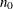and NMC=n, the number of samples kept is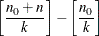where [a] represents the integer part of the number a. The default is THINNING=1.